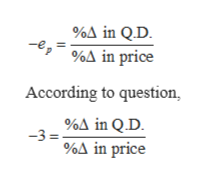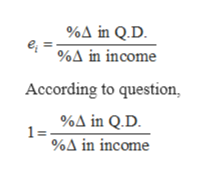# Imagine you work as an economist for a particular airline (A).  Your job entails estimating the passenger demand for airline travel provided by A.  Accordingly, you estimate the following: Price elasticity of demand for A’s service = 3Cross elasticity of demand for A’s service (with respect to airline B’s price) = 2Income elasticity of demand for A’s service = 1 Making sure to show all of your work, if consumer income falls by 5% (due to a recession), and at the same time airline B lowers its price by 10%, all else equal, what would you specifically recommend A due to its price to maintain its quantity of passengers (i.e., lower or raise its price and by what percent)?  Hint:  Elasticities are ratios of percentage changes.

Question
101 views

Imagine you work as an economist for a particular airline (A).  Your job entails estimating the passenger demand for airline travel provided by A.  Accordingly, you estimate the following:

Price elasticity of demand for A’s service = 3

Cross elasticity of demand for A’s service (with respect to airline B’s price) = 2

Income elasticity of demand for A’s service = 1

Making sure to show all of your work, if consumer income falls by 5% (due to a recession), and at the same time airline B lowers its price by 10%, all else equal, what would you specifically recommend A due to its price to maintain its quantity of passengers (i.e., lower or raise its price and by what percent)?  Hint:  Elasticities are ratios of percentage changes.

check_circle

Step 1

Price elasticity of demand (ep) can be formulated as % change in quantity demanded of a good due to the % change in its price. As it is known that the price of a good and quantity demanded are negatively related to each other, the formula for price elasticity is:help_outlineImage Transcriptionclose%A in Q.D %A in price According to question %A in Q.D -3= %A in price fullscreen
Step 2

Income elasticity of demand (ei) can be formulated as % change in quantity demanded of a good due to the % change in the income of a consumer. As it is known that income of a consumer and the quantity, he demands is positively related to each other, the formula for income elasticity is:help_outlineImage Transcriptionclose%A in Q.D. %A in income According to question, %A in Q.D. 1 = %A in income fullscreen
Step 3

Now, if the income of the consumer falls by 5%, he will demand l...

### Want to see the full answer?

See Solution

#### Want to see this answer and more?

Solutions are written by subject experts who are available 24/7. Questions are typically answered within 1 hour.*

See Solution
*Response times may vary by subject and question.
Tagged in